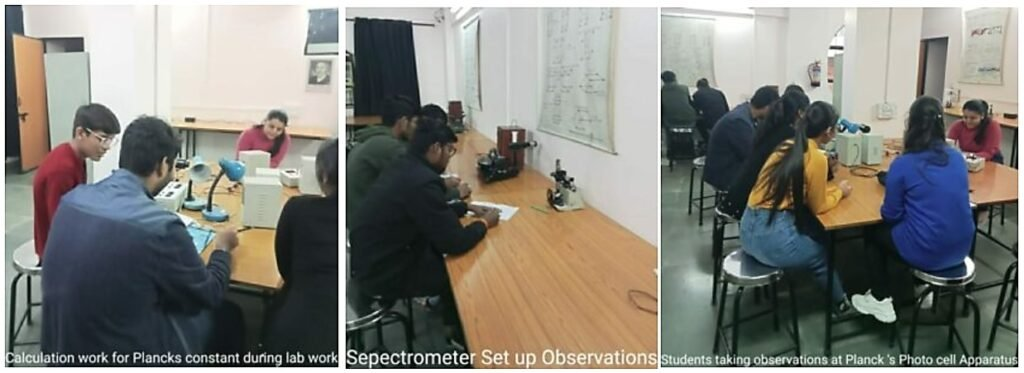B001

• Lab Incharge                  –   Dr. ANIL KUMAR

• Lab Attendant                —  Mr. SUHAN SINGH

• Subjects                         –   Applied Physics – I & II

In Applied science department, Physics lab is well equipped with all the modern gadgets and instruments. Each practical batch comprises of 20 students with two students working together on one set of equipment.
The laboratory manuals and the list of experiments have been displayed in the lab.

• 1st Semester – 10 experiments (10 sets of experiments are in running condition)
• 2nd Semester – 10 experiments (08 sets of experiments are in running condition)Experiment List for the Semester-I

1. To determine the wave length of Sodium light by Newton’s ring.

2. To determine the wave length of Sodium light by Fresnel’s biprism.
3. To determine the wave length of Sodium light by diffraction grating.
4. To determine the refractive index of prism using spectrometer.
5. To find the dispersive power of prism using spectrometer and mercury source.
6. To determine specific rotation of cane sugar solution with the help of half shade polarimeter.
7. To find the wavelength of He-Ne laser using transmission diffraction grating.
8. To determine the numerical aperature (NA) of an optical fibre.
9. To plot the graph between the distance of the knife-edge from the centre of the gravity and the time period of bar pendulum. From the graph find
A)  acceleration due to gravity.
B)  the radius of gyration and the moment of inertia of the bar about an axis.
10. To determine the velocity of ultrasonic waves using an ultrasonic spectrometer in a given liquid (kerosene oil).
11. To verify inverse square law by photo conducting cell.
12. To determine planck’s constant.

Experiment List for the Semester-II

1. To determine the e/m ratio of an electron by J.J.Thomson method.
2. To measure the frequency of a sine wave voltage obtained from signal generator and to obtain Lissajous pattern on the CRO screen
by feeding two sine wave signals from 2 signal generators.
3. To determine the frequency of ac mains using sonometer .
4. To determine the frequency of electrically maintained tuning fork by Melde’s method .
5. Computer simulation (simple application of Monte carlo) Brownian motion, charging and discharging of a capacitor.
6. To study the charging and discharging of capacitor and also to find out the time constant.
7. To study the Hall effect .
8. To verify the Stefan’s law.
9. To determine the energy band gap of a semiconductor by 4-probe method.
10. To study the I-V characteristics of zener diode.
11. To find the thermal conductivity of the poor conductor by Lee’s disk method .
12. To study the thermo emf using thermocouple and resistance using Pt resistance thermometer.